# Electric field of charge distributions

## Homework Statement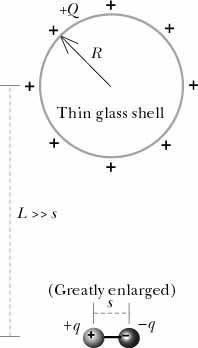A small, thin, hollow spherical glass shell of radius R carries a uniformly distributed positive charge +Q, as shown in the diagram above. Below it is a horizontal permanent dipole with charges +q and -q separated by a distance s (s is shown greatly enlarged for clarity). The dipole is fixed in position and is not free to rotate. The distance from the center of the glass shell to the center of the dipole is L.

The charge on the thin glass shell is +6e-09 coulombs, the dipole consists of charges of 4e-11 and -4e-11 coulombs, the radius of the glass shell is 0.15 m, the distance L is 0.45 m, and the dipole separation is 2e-05 m. Calculate the net electric field at the center of the glass shell. The x axis runs to the right, the y axis runs toward the top of the page, and the z axis runs out of the page, toward you.

E^^->_(net) = < , , > N/C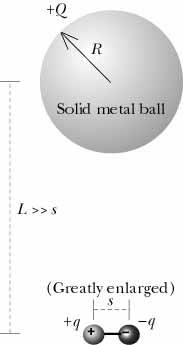If the upper sphere were a solid metal ball with a charge +6e-09 coulombs, what would be the net electric field at its center?
E^^->_(net) = < , , > N/C

Which of the diagrams below best shows the charge distribution in and/or on the metal sphere?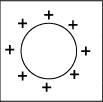A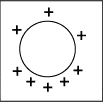B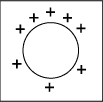C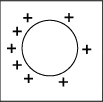D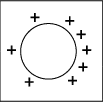E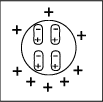F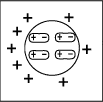G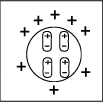H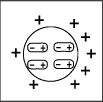J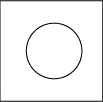K

## Homework Equations

Electric Force of Dipole: (1/4*pi*epsilon_0)(q*s)/r^3
Electric Force of Sphere: (1/4*pi*epsilon_0)(Q/r^2)

## The Attempt at a Solution

The question is kind of confusing to me, as it asks to calculate the net electric field at the CENTER of the glass shell. Right now I'm assuming that the electric field AT THE CENTER of the glass shell is zero (is this assumption not correct? since r<R inside the sphere) and that to answer this first part of the question, it would just be the electric field of the DIPOLE, which is <.000079,0,0>N/C.
Also, if I do calculate the electric field of the glass shell, from the center of the glass shell to the center of the dipole, whose distance is .45m, then I would obtain <0,266.667,>N/C.

As for the second part, I would assume it would be the same values as when I calculate the net electric field at the center of the glass shell.

For the last part (multiple choice) I'm not entirely sure, but I'm leaning towards C.

HELP WOULD BE MUCH APPRECIATED, and thank you in advance.